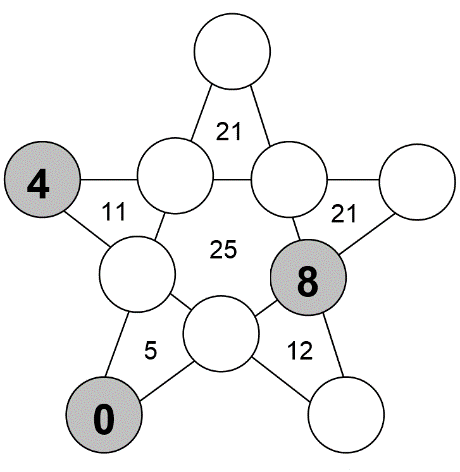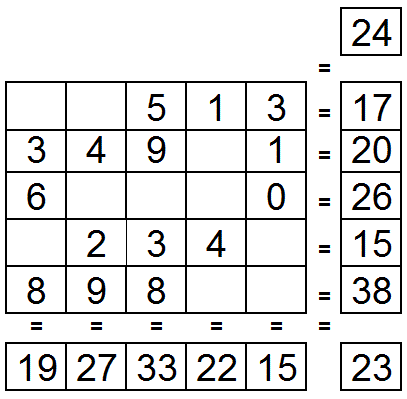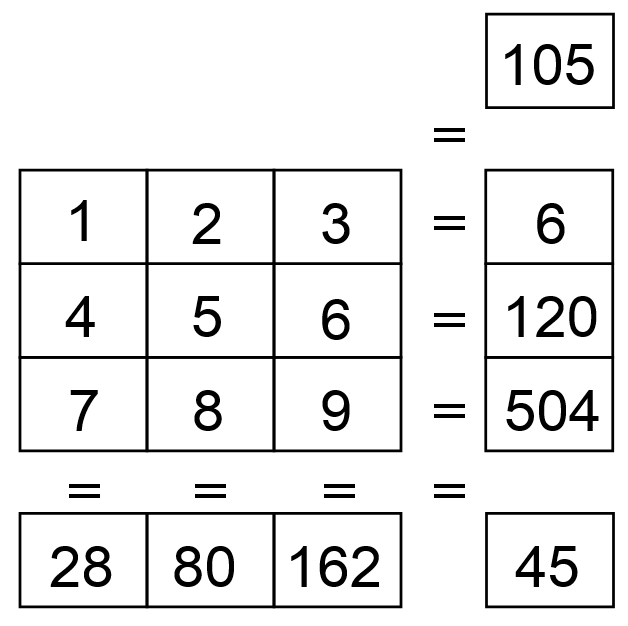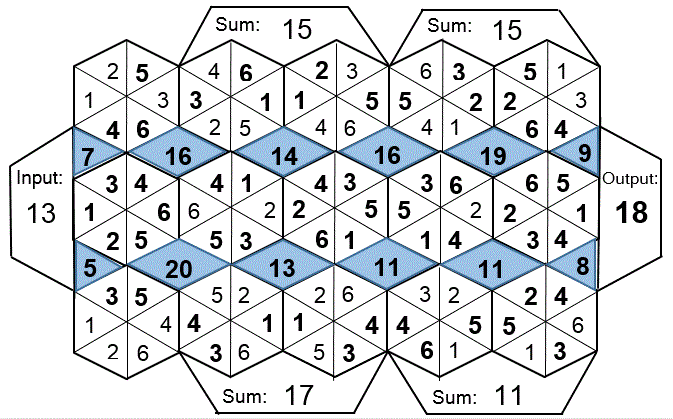Select Page

Each month, a new set of puzzles will be posted.  Come back next month for the solutions and a new set of puzzles, or subscribe to have them sent directly to you.

## MIND-Xpander Logic Problem

Can you determine their ages? – Tatum is nine years older than Ryan and Ryan is four years older that Charley Rose. Mia is three years older than Jayden and one year younger than Tatum. Jordan is one year younger than Kylee Rae and Kylee Rae is five years older than Ryan. Dylan is three years younger than Jake and the same age as Jordan. The sums of Kylee Rae’s and Jordan’s ages is 21.

## STAR-Sums Puzzles

The number within the five triangles of the star is equal to the sum of the three numbers in its surrounding circles. The number in the centre of the star is equal to the sum of the five numbers in its surrounding circles. The numbers in the circles can only be 0 to 9 and each number can be used only once. Three numbers have been provided to get you started. Find the remaining 7 numbers.## SQUARE-UP Puzzles

The missing numbers are between 0 & 9. The numbers in each row add up to the totals on the right, and the columns add up to the totals along the bottom. The sums of the diagonals are also given. Find the missing numbers? (Note: there may be more than one solution.)## Feedback

There are more than one way of doing these puzzles and may well be more than one answer.  Please let me and others know what alternatives you find by commenting below.  We also welcome general comments on the subject and any feedback you'd like to give.

If you have a question that needs a response from me or you would like to contact me privately, please use the contact form.

## Get more puzzles!

If you've enjoyed doing the puzzles, consider ordering the book; 150+ of the best puzzles in a handy pocket sized format. Click here for full details.

## MIND-Xpander Logic Problem

1. Ship Ahoy – A ship is at anchor. Over its side hangs a rope ladder with rungs a foot apart. The tide rises at the rate of 8 inches per hour. If the ladder was 8 feet above the water level when the tide began to rise, how much of the rope ladder will remain above water after 6 hours?

Solution: Since the ship is afloat, the water level in relation to the ship is always the same. Therefore, the rope ladder is still eight feet above the water level.

2. Water lilies on a certain lake double in area every twenty-four hours. From the time that the first water lily appears until the lake is completely covered takes 60 days. On what day is the lake half-covered?

Solution: The lake is half-covered on the 59th day. Since the water lilies double each day, the lake is half-covered the day before it is fully covered. (Sometimes it’s easier to solve a problem backward)

## EQUATE-Times Puzzles

The missing numbers are between 1 & 9 with no repests. The three numbers in each row, when multiplied or times together, equals the totals on the right, and the columns, when multiplied together, equals the totals along the bottom. The multiples of the two diagonals are also given. Solve the puzzle by finding all of the missing numbers that satisfy all of the multiplied results as shown.

Solutions:## HEXAGON-numeric Puzzle (Level 3)

Fit the numbers 1 – 6 in each hexagon and where the hexagon sides touch each other, the numbers in these 2 segments will be the same. No number can be repeated in a hexagon. The numbers in the shaded areas are the sum of the numbers in the 2 or 4 segments that they side with. The numbers in the ‘Input’ and ‘Output’ boxes are the sum of the numbers in the 3 triangular segments that they side with. The numbers in the ‘Sum’ boxes are the sum of the numbers in the 4 segments that they side with. Solve the puzzle and find the Output’ number (?).

Solution: